# 6 kinds of common power supply design circuit introduction

The rapid advancement of electronic information technology has accelerated progress in the field of power supply technology, presenting power supply engineers and technicians with unprecedented opportunities and challenges, ranging from household appliances to large-scale instruments and equipment used in the power industry. Power supply to give energy, which necessitates a significant number of engineers with experience in power supply to complete the design and development.

As a licensed power engineer, gaining job experience is critical, but you should also work on improving your theoretical knowledge. You might be able to use some regularly used power circuits if you save them.

Flyback Power Supplies using Ferrite Amplifiers When using a dual output flyback power supply that gives real power at both outputs (5V 2A and 12V 3A, both regulated to within 5%), When the voltage exceeds 12V, it enters a zero-load state and is no longer able to be regulated within the 5% limit. Although linear regulators are a possible option, they are still not ideal because they are costly and inefficient.

Even in a flyback topology, we recommend using a magnetic amplifier at the 12V output. A ferrite magnetic amplifier is recommended to save money. The control circuit of the ferrite magnetic amplifier.  on the other hand, differs from that the traditional rectangular hysteresis loop material (high permeability material). To keep power at the output, the ferrite's control circuitry (D1 and Q1) sinks current. This circuit has undergone extensive testing. The transformer windings are rated for output voltages of 5 and 13 volts. Even with less than 1W input power (5V 300 mW and 12V zero loads), the circuit can achieve 12V output 5% regulation.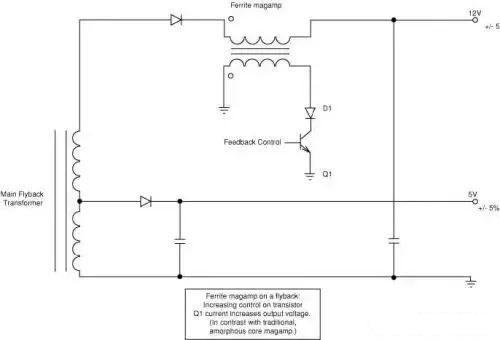Figure. 1

02

Protection Against Overcurrent Taking Advantage of Existing Crowding Circuits Consider flyback power supply with 5V 2A and 12V 3A outputs. When the 12V output achieves no load or a very light load, one of the important specifications of this power supply is to provide overpower protection (OPP) for the 5V output. There is a 5% voltage control requirement on both outputs.

The use of a sensing resistor in the standard approach impairs cross-regulation performance, and the fuse is not inexpensive. For overvoltage protection, there are now arc suppression circuits (OVP). The circuit can meet both OPP and voltage regulation criteria at the same time, and this function can be accomplished with the help of a partial arc suppression circuit.

R1 and VR1 provide an active dummy load at the 12V output, as shown in Figure 2, allowing for 12V voltage regulation even when the 12V output is weakly loaded. The voltage on the 5V output will decline when the 5V output is in an overload condition. A dummy load will consume a significant amount of current. The voltage drop across R1 can be used to detect this large amount of current. Q1 turns on and triggers the OPP circuit.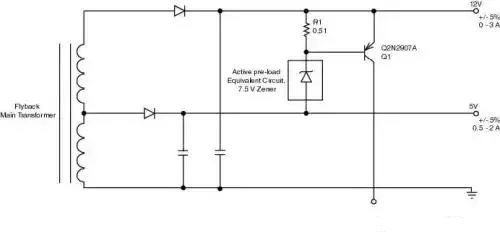Figure. 2

03

Flyback is the most common topology in active shunt regulators and dummy load line voltage AC  to low voltage DC switching power supply equipment. The unique cost-effectiveness of supplying numerous output voltages simply by adding additional windings to the transformer secondary is one of the key reasons for this.

The feedback is usually provided by the output with the strictest output tolerance requirements. The turns per volt for all other secondary windings is then determined by this output. The required output voltage cross-regulation is not always attained at the outputs due to leakage inductance effects, especially if a given output is unloaded or very lightly loaded while the other outputs are fully loaded.

Under such circumstances, a post-regulator or dummy load can be utilized to keep the output voltage from rising. However, due to their higher cost and decreasing efficiency, post-regulators or dummy loads are not as appealing as they once were, especially in recent years for no-load and/or standby input power consumption in a range of consumer applications. As restrictions become more stringent, the design is beginning to lose favor. Figure 3 shows an active shunt regulator that not only solves the problem of regulation but also reduces costs and improves efficiency.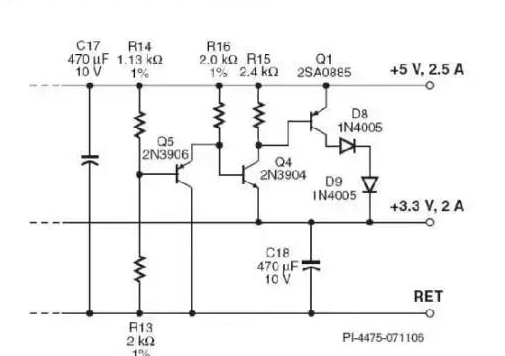Figure. 3 Active Shunt Regulator for a Multiple-Output Flyback Converter

The following is how the circuit works: Resistor dividers R14 and R13 bias transistor  Q5, which keeps  Q4  and Q1 off when both outputs are regulated. The current flowing through  Q5 functions as a modest dummy load at the 5V output under these operating conditions.

The difference between the 5V and 3.3V outputs is typically 1.7V. When a load requires more current from the 3.3V output and the load current from the 5V output does not increase by the same amount, the 5V output's output voltage rises in comparison to the 3.3V output's voltage. Q5 will be biased off since the voltage difference is more than 100 mV, turning on  Q4 and Q1 and letting current flow from the 5V output to the 3.3V output. The voltage at the 5V output will be reduced as a result of this current, lowering the voltage differential between the two outputs.

The difference in voltage between the two outputs determines the amount of current in Q1. As a result, even in the worst-case scenario of a full load on the 3.3V output and no load on the 5V output, the circuit keeps both outputs in regulation. Because the VBE temperature fluctuations in each transistor can cancel each other out, Q5 and Q4 in the design provide temperature compensation. The diodes D8 and D9 are optional, although they can be utilized to reduce power dissipation in Q1 and eliminate the requirement for a heat sink.

Under full and light load conditions, the circuit is mostly inefficient because it simply reacts to the relative difference between the two voltages. The active dissipation of this circuit can be lowered by 66 percent when compared to a grounded shunt regulator since the shunt regulator is connected from the 5V output to the 3.3V output. As a result, efficiency is great at full load and power consumption is low from light to no load.

04

Switching Power Supplies with High-Voltage Input StackFETs are a type of transistor that can be used in a variety of applications An auxiliary power stage that can deliver steady low-voltage  DC to analog and digital circuits is frequently required in industrial equipment that operates on three-phase AC. Industrial drives, UPS  systems, and energy meters are examples of such uses.

The requirements for such power supply are far more demanding than those for conventional switches purchased off the shelf. Not only are the input voltages greater in these applications, but the equipment built for three-phase applications in industrial environments must be able to withstand a wide range of variations, such as lengthy dip durations, power surges, and the loss of one or more phases on occasion. Furthermore, the input voltage range of such auxiliary power sources might be anywhere from 57 to 580 VAC.

Due to the high cost of high-voltage MOSFETs and the dynamic range constraints of standard PWM control loops, designing such a wide-range switching power supply might be difficult. StackFET technology enables the use of low-cost, low-voltage MOSFETs rated at 600V with a Power Integrations integrated power controller to create simple, low-cost switching power supplies that can operate across a wide input voltage range.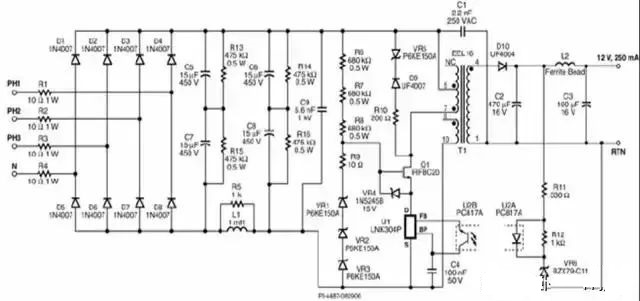Figure. 4 Three-Phase Input 3W Switching Power Supply using StackFET Technology

The circuit operates as follows: the circuit's input current can originate from a three-phase three-wire or four-wire system, as well as a single-phase system. The diodes D1-D8 make up the three-phase rectifier. Inrush current is limited by resistors R1-R4.

Resistors R13 and R15 are used to balance the voltage across the input filter capacitors.

The source of Q1 will be pulled low when the MOSFET inside the integrated switch (U1) comes on, R6, R7, and R8 will give gate current, and the junction capacitance of VR1 to VR3 will turn on Q1. The gate-source voltage delivered to Q1 is limited by the Zener diode VR4. When the MOSFET in U1 is turned off, a 450 V clamping network consisting of VR1, VR2, and VR3 clamps U1's maximum drain voltage. U1's drain voltage will be limited to around 450 V as a result of this.

Q1 receives any additional voltage at the end of the coil attached to it. The total rectified input DC voltage and flyback voltage are effectively distributed between Q1 and U1 with this configuration.

Clamp network  VR5, D9, and R10 are used to restrict the peak voltage on the primary owing to leakage inductance during the flyback interval, while resistor R9 is utilized to control high frequency oscillations during switching.

D1 is in charge of output rectification. The output filter is C2. To reduce switching ripple at the output, L2 and C3 form a secondary filter.

VR6 will turn on when the output voltage exceeds the total voltage drop across the optocoupler diode and VR6. Changes in the output voltage affect the current running through the U2 optocoupler diode, which changes the current flowing through the U2B transistor. The following cycle is stopped when this current exceeds the FB pin threshold current of U1. Controlling the number of activate and inhibit cycles can help regulate output. When the switching cycle is started, the current climbs to U1's internal current limit, and the cycle finishes. R11 is used to control the feedback loop's gain and limit the current through the optocoupler during transient loads. The  Zener diode VR6  is biased by resistor R12.

The circuit is protected against loss of feedback signal, short-circuit at the output, and overload by  IC U1  (LNK 304), which has built-in functionalities. There is no need to add an extra bias winding to the transformer because U1 is powered directly from its DRAIN pin. C4 is utilized to decouple the internal power supply.

05

The EMI  filter circuit in the AC/DC converter can be simplified and reduced in cost by using the right rectifier diodes. The EMI filter circuit of the AC/DC converter can be simplified and reduced in cost using this circuit.

A vast number of EMI filter components, such as X and Y capacitors, are required to make an AC/DC power supply EMI compliant. A bridge rectifier is included in all standard AC/DC power supply input circuits to rectify the input voltage (typically 50-60 Hz). Standard diodes such as the 1N400X  series diodes can be utilized because this is a low-frequency AC input voltage, and they are also the cheapest.

To comply with established EMI restrictions, these filter devices are employed to minimize EMI generated by power sources. However, because EMI tests begin at 150 kHz and the AC line voltage frequency is only 50 or 60 Hz, the reverse recovery time of conventional diodes used in bridge rectifiers (see Figure 1) is long and not often connected to EMI generation.

Input filter circuits used to contain capacitors in line with the bridge rectifier to suppress any high frequency waveforms created by the rectification of the low frequency input signal.

If rapid recovery diodes are used in the bridge rectifier, these capacitors are not required. They swiftly recover when the voltage between these diodes begins to reverse (see Figure 2). By decreasing subsequent high frequency turn-off snaps and EMI, this minimizes stray line inductance excitation in the AC input line. Because only two diodes can be turned on per half cycle, only two of the four diodes must be rapid recovery diodes. Similarly, just one of the two diodes that conduct each half cycle must have a quick recovery characteristic.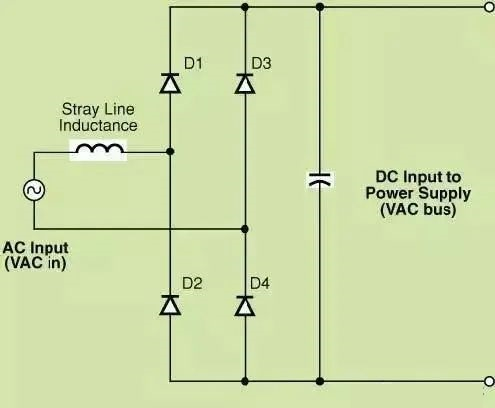Figure. 5 Typical input stage of an SMPS using a Bridge Rectifier on the AC Input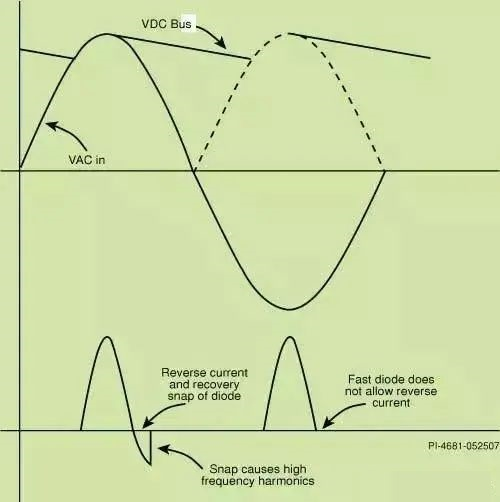Figure. 6 Input Voltage and Current Waveforms Showing Diode Snap at the end of Reverse Recovery

06

Soft-Start Disabling Low-Cost Outputs to Control Current Spikes Some multiplex output power supplies are intended to disconnect the outputs when the standby signal is activated to meet severe standby power criteria.

A series bypass bipolar transistor (BJT) or a MOSFET is typically used to do this. If the power transformer is designed with the additional voltage drop of the transistors in mind, BJTs can be a suitable and less expensive alternative to MOSFETs for low current outputs.

A simple BJT series bypass switch with a voltage of 12 V, an output current of 100 mA, and a big capacitor is shown in Figure 10. (CLOAD). Q1 is a series pass transistor whose switching is controlled by Q2 based on the state of the standby signal. Q1 requires enough base current to function in saturation at minimal beta and maximum output current, hence resistor R1 is rated to ensure that. To manage the transient current at turn-on, PI advises adding an additional capacitor (Cnew). Q1 enters the capacitive load fast after turning on if Cnew is not added, resulting in a high current spike. To handle this transitory spike, Q1's capacity must be raised, resulting in a cost increase.

Current spikes can be eliminated by using Cnew as an additional "Miller capacitor" for Q1. The dv/dt value of Q1's collector is limited by this extra capacitance. Less charging current goes into Cload as the dv/dt value decreases. Specify a capacitor value for Cnew such that the current flowing into R1 is equal to the optimum output dv/dt value of Q1 multiplied by the value of Cnew.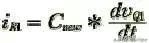Figure. 7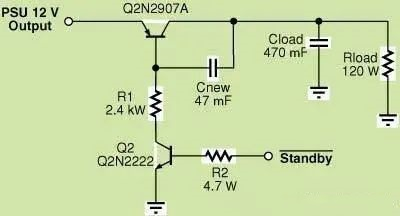Figure. 8 A Simple Soft-Start Circuit can Disable Power Supply Output during Standby while Eliminating Current Spikes during Turn-on. Therefore, a Small Transistor (Q1) can be used to Keep Costs Low

After learning these typical power supply circuits, let's make a simple power supply design circuit by using LM317

## Introduction to LM317

One of the most extensively utilized power integrated circuits is the LM317. It not only features the simplest fixed three-terminal voltage regulator circuit, but it also has adjustable output voltage characteristics. It also boasts a wide voltage regulation range, excellent voltage regulation performance, low noise, and a high ripple rejection ratio. The following are its primary performance indicators.

Maximum input-output voltage difference: 40V DC, minimum input-output voltage difference: 3V DC; use environment Temperature: -10-+85°C; output voltage: 1.25-37V DC; output current: 5mA-1.5A; the chip has overheating, overcurrent, and short circuit protection circuits; maximum input-output voltage difference: 40V DC, minimum input-output voltage difference: 3V DC; use environment Temperature: -10-+85°C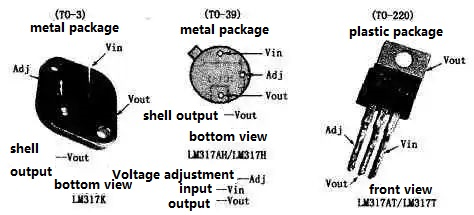Figure. 9

Figure 9 shows the outline and pin arrangement of several commonly used (different package forms) LM317.

Because the voltage between the output terminal (pin 2) and the adjustment input terminal (pin 3) is maintained at 1.25V, the adjustment output can be achieved by adjusting the voltage dividing resistors R1 and R2 connected between the output terminal and ground to change the potential of the ADJ terminal. The voltage serves the following purpose, as shown in Figure 10:

The 1.25V constant voltage across R1 generates a constant current that passes through R1 and R2, with the voltage created on R2 being applied to the ADJ terminal. At this point, the output voltage Vo is determined by the R1:R2 ratio. When R2's resistance rises, the output voltage rises, as follows:

Vo=1.25[(R1+R2)/R2].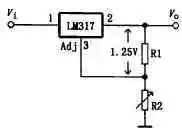Figure. 10

Figure 11 depicts the schematic diagram. The output voltage can be constantly adjusted between 1.25 and 37V by changing the R1 and R2 ratio.

V1 and V2 have the following functions: when the output is short-circuited, V2 discharges the voltage on C2 to achieve reverse bias protection. When the input is short-circuited, the voltage stored on components like C3 is discharged through V1, preventing the internal adjustment tube from becoming reversed biased. C2 is used to boost the IC's ripple rejection capacity. C3 is used to boost the IC's transient response. For input rectification filtering, C1 is utilized. When a large current is output, the IC will be cut off due to the high temperature rise, and a heat sink with an appropriate area must be added. R2 should use a linear potentiometer.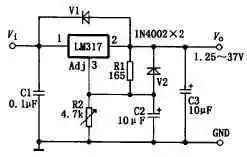Figure. 11

## 1.25-120V maintenance and experimental power supply

Figure 12 depicts the schematic diagram. The circuit consists of four LM317s, and R2 is the single variable that controls the output potentials of the four groups. Adjust R2, and the output potential of IC4 is continually variable between 1.25-30V, while the output potential of IC1-IC3 linked in series with it changes as well, resulting in four sets of DC stable voltages ranging from 1.25 to 120V.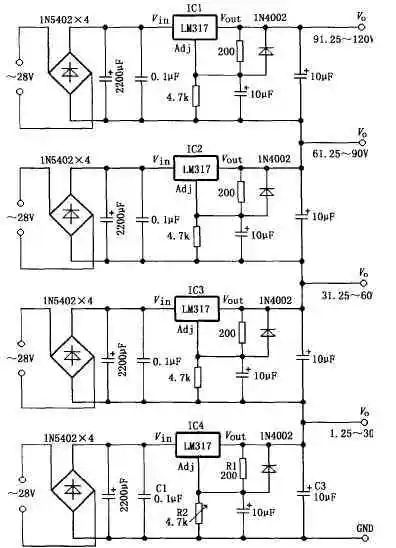Figure. 12

## Slow start 15V power supply

The schematic diagram is shown in Figure 13. The output voltage Vout charges C2 through R1 and V1.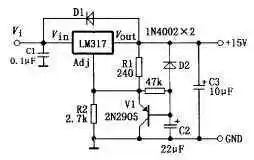Figure. 13

V1 is saturated and turned on at the start, and Vout is the lowest (about 1.5V). V1 eventually exits saturation and tends to be cut off as the voltage on C2 rises, and Vout rises to the rated value. The soft-start time can be altered by altering the R1 and C2 constants. After shutdown, D1 is utilized to swiftly discharge the charge on C2. The value of the output voltage Vout can be modified by adjusting the value of R2. The settings in the figure have a 15V output voltage. 9012 can be used in place of V1 in the diagram.

## TTL level controlled 5V power supply

Figure 14 depicts the schematic diagram. The output voltage is 5V when the external TTL  control signal causes V1 to cut off. Change the value of R2 to get a variety of voltage outputs. An NPN tube, such as the 9013, can be used to replace V1.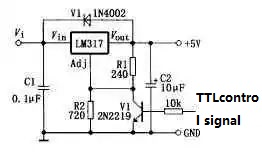Figure. 14